in StemSociallast year

HELLO Hiveans

I will be talking about indices in mathematics today, so many find it difficult but after today, it will be a walkaway topic.

INDICES.

Index are an expression of an equation that has a base and power/index.
It is the opposite of a logarithm.
Mathematically: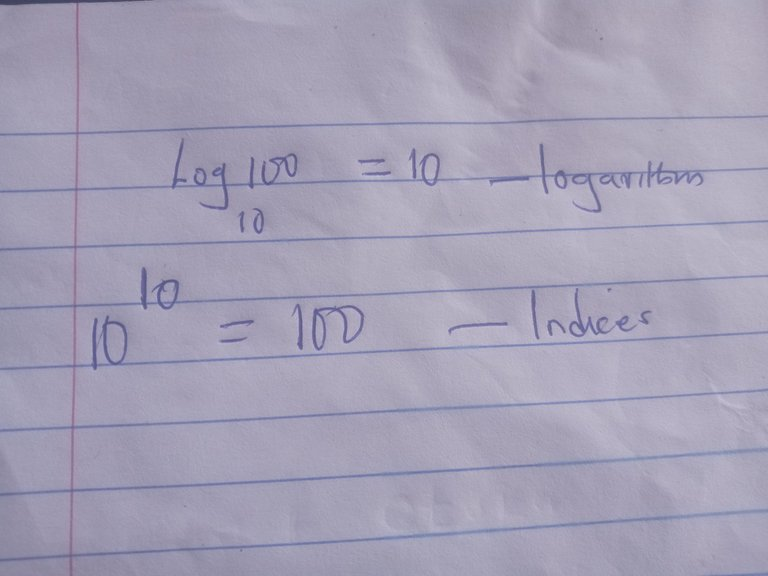LAW OF INDICES

1. Multiplication law of indices:

This law state that when two or more equation or expression of the same base are joined by a multiplication(×) sign, You take one base and add the powers.

Mathematically:

3^(6)× 3^(4)
=3^(6-4)
3^2.

1. Division law of indices:

This law states that when two equations or expressions are joined by the division sign(÷), u takes one base and subtracts the powers.

Mathematically:

10^4 ÷10^2= 10^(4-2)
=10^2.

1. Zero law of indices:

This law state that when an equation or expression is raised to a power of zero(0), The resultant answer is zero(0).

NB:if an alphabet or number is raised to a power of zero, it is equal to zero.

Mathematically:

10^(0)=0

(A)^(0)=0

(3A)^(0) =0.

1. Power law of indices:

This law states that when an equation or expression is raised to a number, all raised to a power of a certain number, You take the base and multiply the powers.

Mathematically:

(2^2)^2= (2)^(2×2)
2^(4)

(2a)^(2)
=4A^2.

1. Fraction law of indices:

This law states that when an expression or equation is raised to the power of a fraction, u takes the denominator as the root and the numerator as the power.

Mathematically.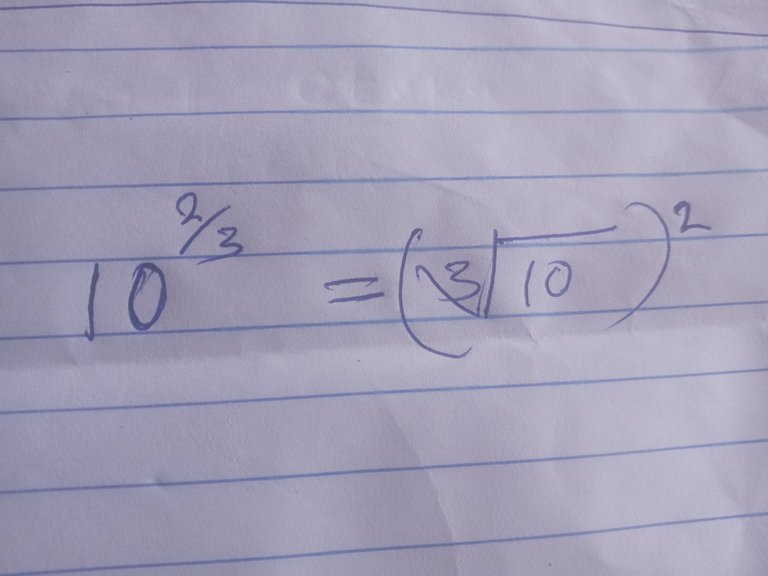Example 1.

Find the expression of the equation below.

(2a)^3 ×(4a)^2
Solution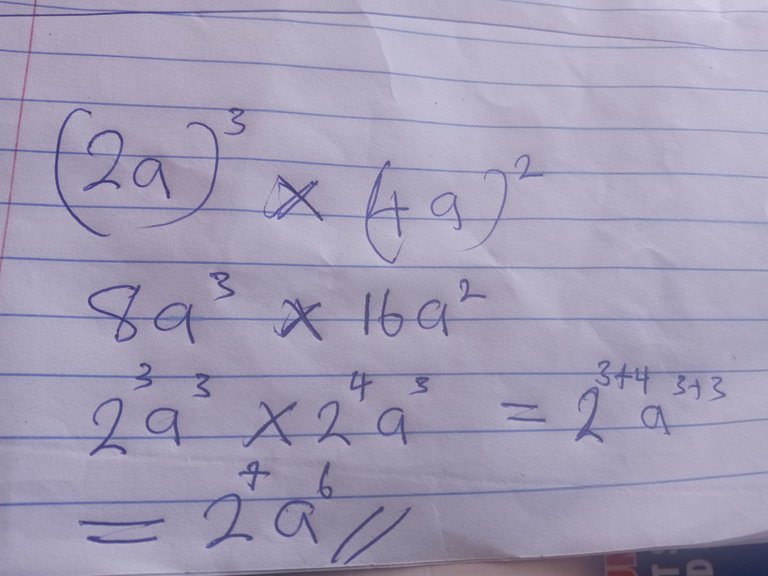Example 2.

Find the expression on the equation

3^4÷9^3
Solution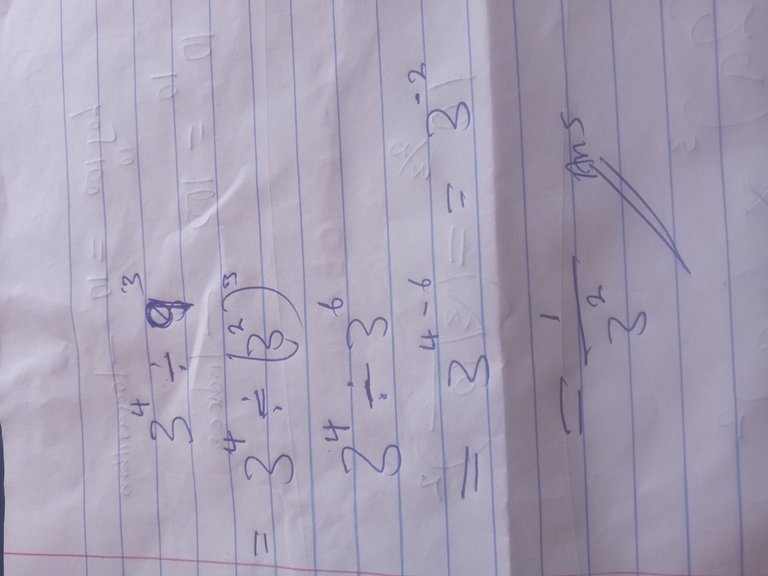Sort:

Congratulations @apehjames! You have completed the following achievement on the Hive blockchain and have been rewarded with new badge(s):You received more than 300 upvotes.Your next target is to reach 400 upvotes.

If you no longer want to receive notifications, reply to this comment with the word `STOP`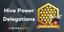Our Hive Power Delegations to the September PUM Winners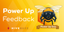Feedback from the October 1st Hive Power Up Day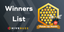Hive Power Up Month Challenge 2022-09 - Winners List